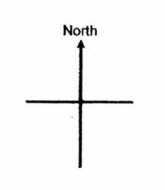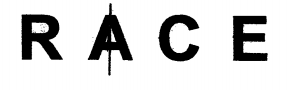# Primary 5 CA1 Practice #4

Time elapsed:
Q1. In 9 586 730, the value of the digit 9 is ____.     [1 mark]
Q2. Ellie spent 16 of her money on a dress and 13 of it on a wallet. What fraction of her money did she spend?     [1 mark]
Q3. Find the value of 59 - 13     [1 mark]
Q4. Wendy was facing north-east after making an anti-clockwise 225° turn. At which direction was she facing at first?     [2 marks]Q5. The common multiples of two 1-digit numbers are 16, 24 and 40. What is the largest possible values of these two 1-digit numbers?     [2 marks]
Q6. A number when rounded to the nearest thousand is 900 000. What is the number?     [1 mark]
Q7. Which of the following has the same value as 11 fifths?     [1 mark]
Q8. Find the value of 37 + 3 x (15 hundreds ÷ 3 tens).     [2 marks]
Q9. Grace, Sandy and Abigail shared some money. The total amount of money Grace and Abigail received was \$1250. The total amount of money Grace and Sandy received was \$830. Abigail's amount of money was four times as much as Sandy's amount of money. How much did Grace receive?     [2 marks]
Q10. How many of the letters have at least a line of symmetry?     [1 mark]Q11. Find the value of 4500 x 20 ÷ 300.     [1 mark]
Q12. Which one of the following fractions is greater than 12 but smaller than 34?     [2 marks]
Q13. Alex spent 25 of his money on transport and 13 of it on food. What fraction of his money had he left?     [2 marks]
Q14. Wendy had 23 of a bar of chocolate. She ate 12 of it. What fraction of the bar of chocolate did she eat?     [1 mark]
Q15. Express 811 as a decimal corrected to 2 decimal places.     [1 mark]
Q16. Wendy had enough money to buy either 8 pears or 12 oranges. She spent all her money on these 2 types of fruits. If she bought 6 pears, how many oranges did she buy?     [2 marks]